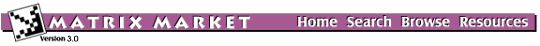## PLATZ: Platzman's oceanographic models

### from the Harwell-Boeing Collection

Set PLATZ
Source: John Lewis, Boeing Computer Services, Seattle, Washington
Discipline: Oceanic modeling
Accession: Summer 1975

The matrices with prefix PLAT are well known as difficult sparse symmetric eigenproblems. The larger matrix is a finite-difference model for the shallow wave equations for the Atlantic and Indian Oceans. The smaller matrix corresponds roughly to the North Atlantic Ocean. The original matrix is derived as the (negative) square of a purely imaginary skew-symmetric matrix. Hence, the eigenvalues occur in pairs (except for an isolated singleton at zero). A list of all of the eigenvalues of the smaller problem are given in the third reference. The matrices with prefix PLSK are the skew-symmetric matrices whose squares are the first two matrices.

The references describe the difficulties encountered in computing the desired eigenvalues in the mid 1970's. The problem is difficult because the desired natural modes correspond to interior eigenvalues of the matrices. The original problem was to find all eigenvalues in (.0001, .024), corresponding to natural modes that could contribute to global tides. The eigenvalues in (.000025, .0001) were also of interest, but were impossible to compute at that time. Modern shift and invert eigenvalue algorithms have little difficulty with these problems if they are capable of handling multiple eigenvalues. The unusual distribution of eigenvalues has, however, played havoc with some eigenvalue codes.

## Matrices in this set:

The Matrix Market is a service of the Mathematical and Computational Sciences Division / Information Technology Laboratory / National Institute of Standards and Technology.

[ Home ] [ Search ] [ Browse ] [ Resources ]